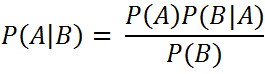`  I request GUEST WRITERS for this web site. Anyone interested please contact me at saravu2k2013@gmail.com Thanks  `

## Find the correct BCNF decomposition for a given database table

#### Question:

11. Consider the relation R(A, B, C, D, E) with set of functional dependencies F = {A → B, C → D}. Let us assume that R is in 1NF. Which of the following is the correct BCNF decomposition of R?

(a) R1 (A, C, D, E) and R2 (A, B)
(b) R1 (A, B, C, E) and R2 (C, D)
(c) R1 (A, B), R3 (C, D), and R3 (A, C, E)
(d) All of the above.

(c) R1 (A, B), R3 (C, D), and R3 (A, C, E)

Discussion:

It is given that R is in 1NF. To proceed further, we need to find the key of R.
Where to start?
From the given set F of functional dependencies, we would understand that the attributes A, C and E are not present in the RHS of functional dependencies. Hence, we shall start with finding the closure of (ACE). Refer here to know how to find closure of anattribute.

Find (ACE)+
Result = ACE
Result = ABCE (from the functional dependency A → B)
Result = ABCDE (from the FD C → D)
At this stage, result = ABCDE which is equal to R. Hence, ACE is a super key.
Is (ACE) a candidate key?
To check this, we need to find the closure of proper subset of (ACE). If the proper subset does not contain another super key, then ACE is a candidate key.
Proper subset of (ACE) consists of (A), (C), (E), (AC), (CE), and (AE). Find the closure for each component. None of them forms the super key. Hence, (ACE) is the candidate key.

Is R in 2NF?
No. The reason is the partial dependency A → B. So, we need to decompose R using the FD A → B. We shall get relations as follows;
R1 (A, B) – it is in 2NF.
R2 (A, C, D, E) – it is not in 2NF because of the violating FD C → D.
We shall break R2 as follows;
R2 (C, D) – it is in 2NF.
R3 (A, C, E) – it is in 2NF.

Are R1, R2 and R3 are in 3NF?
3NF – Transitive dependencies must not present in a relation.
Neither of R1, R2, and R3 have transitive dependencies. Hence, all these relations are in 3NF.

Are R1, R2 and R3 are in 3NF?
BCNF – the LHS of all FDs should be candidate key.
For R1, A → B is the only FD and A is the candidate key.
For R2, C → D is the only FD and C is the candidate key.
For R3, no FDs. Hence, all attributes (ACE) form the key.
So, R1, R2, and R3 are in BCNF.